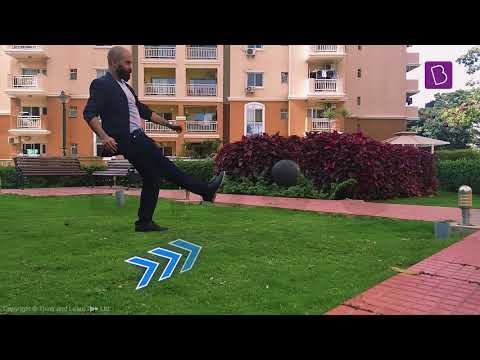# What are the effects of force on an object?

We all might have heard about force. We use the word force daily, and force has become a common word rather than a scientific word.  So let us first know what a force is.

## What is Force?

The push or pull experienced by an object with the mass that causes the velocity is called force. Force is an external agent capable of changing the state of rest or motion of a particular body. It has a magnitude and a direction.

## Formula and SI Unit

The quantity of force is expressed by the product of mass (m) and acceleration (a). Mathematically the equation can be written as

F = ma
Where,

F= Force

m = mass
a = acceleration

When a force acts on an object the objects, the things have different effects of force. So let us know about the impact of force on an object.

## Following are the effects of force on an object

• Force can set a stationary object in motion:

When force is applied to a stationary object, the object tends to move in the direction in which force is applied. For example, applying force on a stationary tennis ball will make it continue its motion in the direction of applied force.

• Force can either stop or slow down the moving object:

Force needs to be applied on a moving object either to stop it or to slow down the object. For example, the moving ball can be stopped by applying external force.

• Force is applied to change the direction of moving object:

The direction of a moving object can be changed by applying the force on that object. For example, the direction of the moving football can be changed by using force at an angle such that the direction is changed.

• The shape of an object can be changed by applying force:

Consider a circular rod, by hammering the rod, which is using an external force; the rod can be turned into a flat sheet.### Refer more

4 (62)(83)(8)

#### Choose An Option That Best Describes Your Problem

Thank you. Your Feedback will Help us Serve you better.